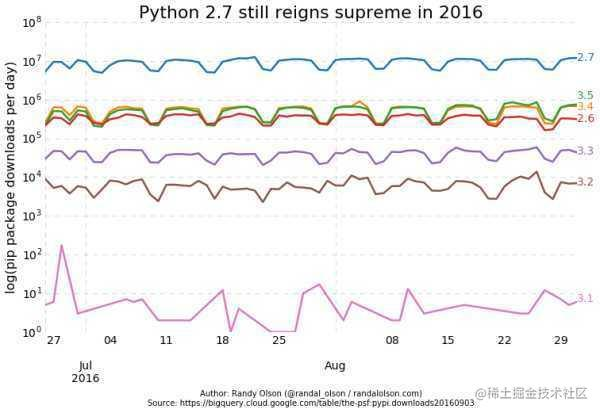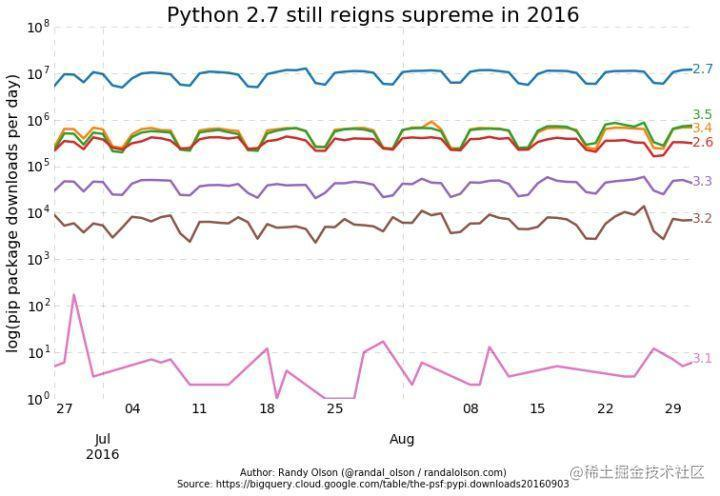Python2和Python3的区别，以及为什么选Python3的原因

• Python 3 才是Python的未来
• Python 官方都建议指直接学习Python 3
• Python 2 只维护到2020年

为什么还要学习Python 2

Python 2只维护到2020年不应该成为你拒绝Python 2的理由

Python官方建议学习Python 3只是一种一厢情愿的行为Python 2的使用量远远超过Python 3。而且，大家注意竖轴的单位，是指数！简单换算一下就知道，仅从下载量来说，Python 2.7的下载量是总下载量的90%！所以，学习Python，想直接抛弃Python 2学习Python 3，几乎是不可能的事情。

• 10% 使用 Python 3
• 20% 既使用Python 2也使用Python 3，Python 2用的更多
• 70% 使用Python 2

Python 2还会存在很长一段时间

stop talking, just do it

• 学习Python前，先了解在Python 3里面已经弃用的Python 2语法，对这些部分简单带过不要花太多时间
• 使用Python 2，不要使用Python 2.7以前的版本
• 使用Python 3，不要使用Python 3.4以前的版本
• 多了解Python 2的future
• 对同一份代码，不要为Python 2和Python 3分别维护分支，努力在一套代码中兼容Python 2和Python 3

print函数:（Python3中print为一个函数，必须用括号括起来；Python2中print为class）

Python 2 的 print 声明已经被 print() 函数取代了，这意味着我们必须包装我们想打印在小括号中的对象。

Python 2

1 print 'Python', python_version()

2 print 'Hello, World!'

3 print('Hello, World!')

4 print "text", ; print 'print more text on the same line'

run result:
Python 2.7.6
Hello, World!
Hello, World!
text print more text on the same line

Python 3

1 print('Python', python_version())

2 print('Hello, World!')

3 print("some text,", end="")

4 print(' print more text on the same line')

run result:
Python 3.4.1
Hello, World!
some text, print more text on the same line

通过input()解析用户的输入：（Python3中input得到的为str；Python2的input的到的为int型，Python2的raw_input得到的为str类型）统一一下：Python3中用input，Python2中用row_input，都输入为str

Python 2
Python 2.7.6
[GCC 4.0.1 (Apple Inc. build 5493)] on darwin

>>> my_input = input('enter a number: ') enter a number: 123 >>> type(my_input)<type 'int'> >>> my_input = raw_input('enter a number: ') enter a number: 123 >>> type(my_input)<type 'str'>

Python 3
Python 3.4.1
[GCC 4.2.1 (Apple Inc. build 5577)] on darwin

>>> my_input = input('enter a number: ') enter a number: 123 >>> type(my_input)<class 'str'>

整除:(没有太大影响)（Python3中/表示真除，%表示取余，//表示地板除（结果取整）；Python2中/表示根据除数被除数小数点位得到结果，//同样表示地板除）统一一下：Python3中/表示真除，%表示取余，//结果取整；Python2中带上小数点/表示真除，%表示取余，//结果取整

Python 2

1 print 'Python', python_version()

2 print '3 / 2 =', 3 / 2

3 print '3 // 2 =', 3 // 2

4 print '3 / 2.0 =', 3 / 2.0

5 print '3 // 2.0 =', 3 // 2.0

run result:
Python 2.7.6
3 / 2 = 1
3 // 2 = 1
3 / 2.0 = 1.5
3 // 2.0 = 1.0

Python 3

1 print('Python', python_version())

2 print('3 / 2 =', 3 / 2)

3 print('3 // 2 =', 3 // 2)

4 print('3 / 2.0 =', 3 / 2.0)

5 print('3 // 2.0 =', 3 // 2.0)
k
run result:
Python 3.4.1
3 / 2 = 1.5
3 // 2 = 1
3 / 2.0 = 1.5
3 // 2.0 = 1.0

xrange模块：

|

python 2.4 与 python 3.0 的比较

......

except    Exception, e :

......

try:

......

except    Exception as e :

......

A bytes object is an immutable array. The items are 8-bit bytes, represented by integers in the range 0 <= x < 256.

bytes 可以看成是“字节数组”对象，每个元素是 8-bit 的字节，取值范围 0~255。

（一）字符串编码(encode) 为 bytes

b = s.encode( 编码方式)

# b 就是 bytes 类型的数据

# 常用的编码方式为 ： "uft-16"    , "utf-8", "gbk", "gb2312", "ascii" , "latin1" 等

# 注 ： 当字符串不能编码为指定的“编码方式”时，会引发异常

(二) bytes 解码(decode)为字符串

s = "张三abc12"

b = s.encode( "gbk")    # 字符串 s 编码为 gbk 格式的字节序列

s1 = b.decode("gbk")   # 将字节序列 b以gbk格式 解码为字符串

# 说明，当字节序列不能以指定的编码格式解码时会引发异常

(三）使用方法举例

coding=gbk

f = open("c:\1234.txt", "wb")
s = "张三李四abcd1234"

-------------------------------

b = s.encode("gbk")
f.write( b )
f.close()

input("?")

coding=gbk

f = open("c:\1234.txt", "rb")
f.seek(0,2) #定位至文件尾
n = f.tell() #读取文件的字节数
f.seek(0,0) #重新定位至文件开始处

------------------------------

s = b.decode("gbk")
print ( s )

------------------------------

f.close()
input("?")

(四) bytes序列，一但形成，其内容是不可变的

s="ABCD"

b=s.encode("gbk")

print b # 显示 65

b = 66

执行该句，出现异常: 'bytes' object does not support item assignment

python 2.4.2以前

chr( K ) 将编码K 转为字符，K的范围是 0 ~ 255

ord( c ) 取单个字符的编码, 返回值的范围: 0 ~ 255

python 3.0

chr( K ) 将编码K 转为字符，K的范围是 0 ~ 65535

ord( c ) 取单个字符的编码, 返回值的范围: 0 ~ 65535

python 2.4.2以前

10/3 结果为 3

python 3.0

10 / 3 结果为 3.3333333333333335

10 // 3 结果为 3

(一） 初始化

a = bytearray(   10 )

# a 是一个由十个字节组成的数组，其每个元素是一个字节，类型借用 int

# 此时，每个元素初始值为 0

（二） 字节数组 是可变的

a = bytearray(   10 )

a = 25

# 可以用赋值语句更改其元素，但所赋的值必须在 0 ~ 255 之间

(三) 字节数组的切片仍是字节数组

(四) 字符串转化为字节数组

#coding=gbk

s ="你好"

b = s.encode( "gbk")     # 先将字符串按某种“GBK”编码方式转化为 bytes

c = bytearray( b )          #再将 bytes 转化为 字节数组

也可以写作

c = bytearray( "你好", "gbk")

(五) 字节数组转化为字符串

c = bytearray( 4 )

c = 65 ; c=66; c= 67; c= 68

s = c.decode( "gbk" )

print ( s )

# 应显示： ABCD

(六) 字节数组可用于写入文本文件

coding=gbk

f = open("c:\1234.txt", "wb")
s = "张三李四abcd1234"

-------------------------------

b = s.encode("gbk")

f.write( b )
c=bytearray( "王五","gbk")
f.write( c )
f.close()

input("?")